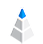# Which Measurement Scale Should We Use?

During statistical observations, we get results we have to present decently. The method of the presentation depends on various factors such as the type of the observed data: discrete or continuous random variables.

The level of measurement or scale of measure is a classification that describes the nature of information within the numbers assigned to variables.

American Psychologist Stanley Smith Stevens developed the most popular classification with four levels: nominal, ordinal, interval, and ratio.

In this article, we will deal with Steven’s classic measurement scales and investigate the categories he described.

## Properties of Measurement Scales

Just before we dive into the topic, we have to understand the different properties of measurement scales. Knowing these can help you to better understand the nature of your variables and data.

### Identity

Each value on the scale has a unique meaning (e.g. 0 false , 1 true)

### Magnitude

There is an ordered relationship between the scale values. Some elements are bigger, and some elements are smaller than others.

### Equal Units

The units are equally divided along the scale. For example, the difference between 2 and 4 is equal to the difference between 6 and 8.

### Location of Zero

Identifies the zero element as minimum value.Now that we know the properties, let us take a closer look at the four different measurement scales.

## Nominal Scale

Nominal is the easiest of all the categories and the lowest measurement level by statistical viewpoint. There are no mathematical relations between the scale values. Sometimes, in the case of nominal scales, we assign numbers to objects as a label. It is not the measure of quantity rather, it measures the identity and difference.## Ordinal Scale

In the case of the ordinal scale, we can order the measurements in terms of “greater than”, “less than” or “equal to”. The ordinal scale is based on rankings. The order matters, but the differences between the variables do not. In the case of ordinal scales, we can use a median, but never use the mean because it is meaningless.

An ordinal scale can be used for placing winners of a race, sizes of clothing and so on.## Interval Scale

The interval scale values have their own identity and magnitude, and the scale units are equal. An interval scale is equally divided along the scale without a predefined zero point.

The zero is not the minimum value of the scale. The difference between the neighboring points are measurable, so the difference in temperature for example between 10° F and 20° F is the same as the difference between 35° F and 45° F.

The most frequently used interval scales are the Celsius scale and the Fahrenheit scale.## Ratio Scale

The ratio scale also has equally spaced units along the scale but with an absolute zero point. Zero is the first element of the scale. This type of scale is widely used in the physical sciences to measure mass, length, duration, and so on.

This scale has all the properties that we described at the beginning of this article. These properties ensure that we execute all the operations, which includes addition, subtraction, multiplication, and division. The absolute zero point allows us to define how many times greater Value A is than Value B.

The Kelvin temperature scale has a true zero point.Tip: AnswerMiner automatically detects data type and helps you to create the perfect visualizations by suggesting the best charts.## Summary Table

In the end, here is a comparison table to better understand the different scaling methods.Connect your data and start the exploration